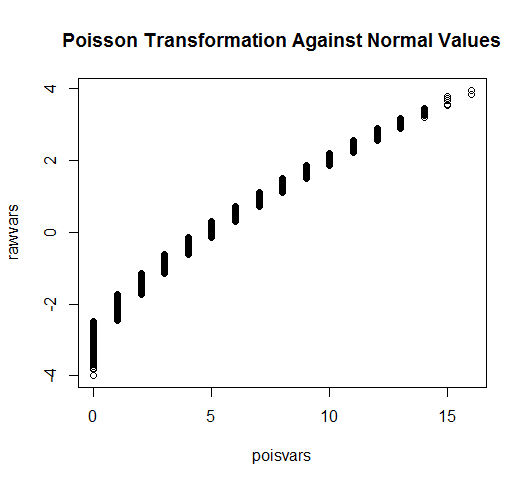## Thursday, February 27, 2014

### Easily generate correlated variables from any distribution

In this post I will demonstrate in R how to draw correlated random variables from any distribution

The idea is simple.
1. Draw any number of variables from a joint normal distribution. 2. Apply the univariate normal CDF of variables to derive probabilities for each variable. 3. Finally apply the inverse CDF of any distribution to simulate draws from that distribution.
The results is that the final variables are correlated in a similar manner to that of the original variables.  This is because the rank order of the variables in maintained and thus correlations are approximately the same though not exact. This methods follows a method I presented in a previous post coded in Stata.  I am not aware of anybody else proposing this method previously.

For example:
```library(MASS)

# We will use the command mvrnorm to draw a matrix of variables

# Let's keep it simple,
mu <- rep(0,4)
Sigma <- matrix(.7, nrow=4, ncol=4) + diag(4)*.3

rawvars <- mvrnorm(n=10000, mu=mu, Sigma=Sigma)

cov(rawvars); cor(rawvars)
# We can see our normal sample produces results very similar to our ```
```#specified covariance levels.

# No lets transform some variables
pvars <- pnorm(rawvars)

# Through this process we already have
cov(pvars); cor(pvars)
# We can see that while the covariances have dropped significantly, ```
```# the simply correlations are largely the same.

plot(rawvars[,1], pvars[,2], main="Normal of Var 1 with probabilities of Var 2") ```
` `
`# Things are looking pretty well so far.  Let's see what happens when we invert `
```#different CDFs.

# To generate correlated poisson
poisvars <- qpois(pvars, 5)
cor(poisvars, rawvars)
# This matrix presents the correlation between the original values generated
# and the tranformed poisson variables.  We can see that the correlation matrix
# is very similar though somewhat "downward biased".  This is because any
# transformation away from the original will reduce the correlation between the
# variables.

plot(poisvars,rawvars, main="Poisson Transformation Against Normal Values")```
` `
```# Perhaps the poisson count distribution is not exotic enough.

# Perhaps a binomial distribution with 3 draws at 25% each
binomvars <- qpois(1-pvars, 3, .25)
# Note, I did 1-p because p is defined differently for the qpois for some ```
```#reason
cor(binomvars, rawvars)

# Or the exponential distribution
expvars <- qexp(pvars)
cor(expvars, rawvars)
# We can see that the correlations after the exponential tranformations are
# significantly weaker (from .7 to .63) but still good representations if
# we are interested in simulating correlations between a normal and exponential
# variables.

plot(expvars,rawvars, main="Exponential Transformation Against Normal Values") ```

```# To make things a little more interesting, let's now transform our probabilities
# into skewed normal distributions.
library(sn)
sknormvars <- qsn(pvars, 5, 2, 5)
cor(expvars, rawvars)

hist(sknormvars, breaks=20) ```
```# Finally in order to demonstrate what we can do let's combine our variables into
# a single matrix.

combvar <- dataframe(sknormvars[,1], poisvars[,2], binomvars[,3], expvars[,4])

cor(combvar)
#          [,1]      [,2]      [,3]      [,4]
#[1,] 1.0000000 0.6853314 0.6826398 0.6256086
#[2,] 0.6853314 1.0000000 0.6748458 0.6402233
#[3,] 0.6826398 0.6748458 1.0000000 0.6325102
#[4,] 0.6256086 0.6402233 0.6325102 1.0000000

# I am going to try to get all of these dirstributions on the same graph
stdcombvar <- t(t(combvar)-apply(combvar,2,min))
stdcombvar <- t(t(stdcombvar)/apply(stdcombvar,2,max))
summary(stdcombvar)

plotter <- data.frame(
values = c(stdcombvar),
rawnorm = rep(rawvars[,1], 4),
type = rep(c("skewed normal",
"poisson",
"binomial",
"exponential"),
each=10000))

library(ggplot2)  ```
```
ggplot(plotter, aes(x=rawnorm ,y=values, color=type)) +
geom_point(shape=1) +     # Use hollow circles
geom_smooth(method=lm,    # Add linear regression line
se=FALSE)  ```
` `

` `
Formatted by Pretty R at inside-R.org

## Thursday, February 6, 2014

### Using MongoHQ to build a Shiny Hit Counter

In serveral previous posts I have posted shiny applications which temporarily store data on shiny servers such as hit counters or the survey tool which I created,  These do not work in the long term since shiny will restart its servers without warning when needed.  In addition, saving data to a shiny server is not an ideal method since special database specific commands should be set up to handle the simultaneous write requirements of web applications.

In this post I will show how to add an effective hit counter to shiny applications using a remote database server (MongoHQ).  Much of my code follows the MongoHQ package demo found at

Start and account with MongoHQ. A Sandbox free database account with 512 MB of memory should be more than sufficient.

Once you have started an account you need to log into app.mongohq.com and start a database as well as a collection.  Within a database you will need to select the admin tab as well in order to create a user id which you can use to log into the collection.

The following code is what I use to create a hit counter.

```# Load the CRAN library
library(rmongodb)

# You can find the host information for the collection under the admin tab.
host <- "myarea.mongohq.com:myport"
db <- "mydatabase"

# Load the collection.  In this case the collection is.
collection <- "OLS-app"
namespace <- paste(db, collection, sep=".")

# Insert a simple entry into the collection at the time of log in
# listing the date that the collection was accessed.
b <- mongo.bson.from.list(list(platform="MongoHQ",
app="counter", date=toString(Sys.Date())))
ok <- mongo.insert(mongo, namespace, b)

# Now we query the database for the number of hits
buf <- mongo.bson.buffer.create()
mongo.bson.buffer.append(buf, "app", "counter")
query <- mongo.bson.from.buffer(buf)
counter <- mongo.count(mongo, namespace, query)

# I am not really sure if this is a good way of doing this
# at all.

# I send the number of hits to the shiny counter as a renderText
# reactive function
paste0("Hits: ", counter)```
Created by Pretty R at inside-R.org

The now database run hit counter can be seen at:
http://econometricsbysimulation.shinyapps.io/OLS-App/

You can find the updated code at github
https://github.com/EconometricsBySimulation/OLS-demo-App/blob/master/server.R